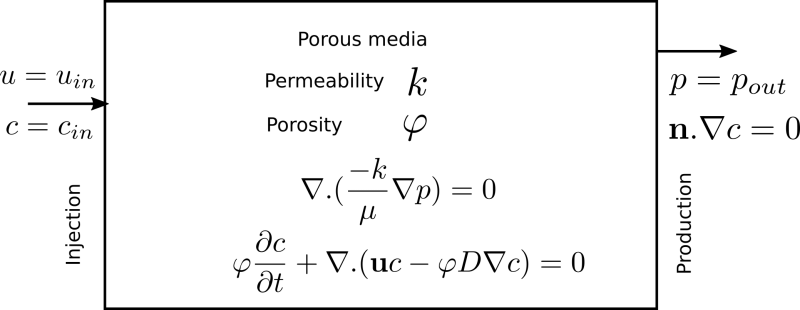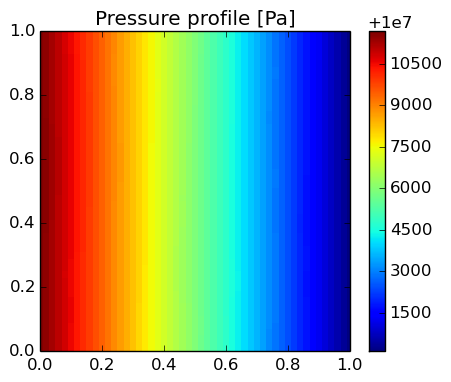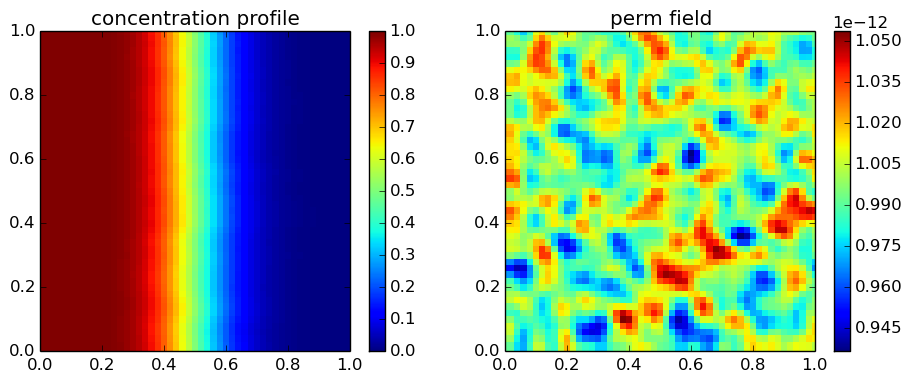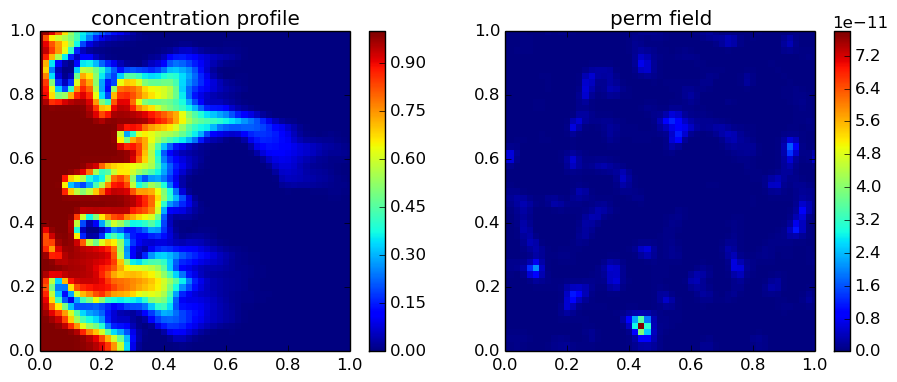# Coupled nonlinear PDEs: two phase flow in porous media

In this post, I'm going to quickly explain how to solve a system of nonlinear equations. In the previous posts (here and here), I explained how to linearize a nonlinear PDE. Now, we are going to linearize two PDEs, which means we need to use the Taylor expansion again, which for a function with two independent variables reads: $$f\left(x,y\right)=f\left(x_{0},y_{0}\right)+\frac{\partial f}{\partial x}\left(x_{0},y_{0}\right)\left(x-x_{0}\right)+\frac{\partial f}{\partial y}\left(x_{0},y_{0}\right)\left(y-y_{0}\right)+h.o.t$$ We are going to use the above equation to linearize the following equations, which describe the two phase flow (oil, and water) in porous media. We ignore the gravity term and assume that the fluids and the porous medium are incompressible: $$\nabla.\left(-k\left(\frac{k_{rw}\left(S_{w}\right)}{\mu_{w}}+\frac{k_{ro}\left(S_{w}\right)}{\mu_{o}}\right)\nabla p\right)=0,$$ and $$\varphi\frac{\partial S_{w}}{\partial t}+\nabla.\left(-\frac{k_{rw}\left(S_{w}\right)k}{\mu_{w}}\nabla p\right)=0.$$ The linearized form, after some algebraic operations, read $$\nabla.\left(-k\left(\frac{k_{rw}\left(S_{w,0}\right)}{\mu_{w}}+\frac{k_{ro}\left(S_{w,0}\right)}{\mu_{o}}\right)\nabla p\right)+\\\nabla.\left(k\left(\frac{\partial k_{rw}\left(S_{w,0}\right)}{\mu_{w}\partial S_{w}}+\frac{\partial k_{ro}\left(S_{w,0}\right)}{\mu_{o}\partial S_{w}}\right)\nabla p_{0}S_{w}\right)=\\\nabla.\left(k\left(\frac{\partial k_{rw}\left(S_{w,0}\right)}{\mu_{w}\partial S_{w}}+\frac{\partial k_{ro}\left(S_{w,0}\right)}{\mu_{o}\partial S_{w}}\right)\nabla p_{0}S_{w,0}\right)$$ and $$\varphi\frac{\partial S_{w}}{\partial t}+\nabla.\left(-\frac{k_{rw}\left(S_{w,0}\right)k}{\mu_{w}}\nabla p\right)+\\\nabla.\left(-\frac{k}{\mu_{w}}\frac{\partial k_{rw}\left(S_{w,0}\right)}{\partial S_{w}}\nabla p_{0}S_{w}\right)=\\\nabla.\left(-\frac{k}{\mu_{w}}\frac{\partial k_{rw}\left(S_{w,0}\right)}{\partial S_{w}}\nabla p_{0}S_{w,0}\right)$$ We will discretize the above equations using the FVTool, which gives us a system of linear equations, i.e., $$\left[\begin{array}{cc} J_{p1}+M_{bc,p} & J_{S_{w}1}\\ J_{p2} & J_{S_{w}2}+M_{bc,S_{w}} \end{array}\right]\left[\begin{array}{c} \mathbf{p}\\ \mathbf{S_{w}} \end{array}\right]=\left[\begin{array}{c} RHS_{1}+RHS_{bc,p}\\ RHS_{2}+RHS_{bc,S_{w}} \end{array}\right]$$ Subscript 1 denotes the first equation (continuity) and 2 denotes the second equation (water saturation balance). Note how we add the boundary condition terms to the equations. I'm not going to explain it in more details, because I'm too tired and it's 1:00 o'clock, but I give you my word that this is the right way to do it! For each time step, the above equation needs to be solved in a loop until the new values of $S_w$ converges to the initial estimates $S_{w,0}$. Let me start the implementation:

In :
% Go to the FVTool folder and initialize the toolbox
cd('/home/ali/MyPackages/FVTool/')
FVToolStartUp()

Out:
AGMG 3.x linear solver is NOT available.
warning: PVTtoolbox could not be found; Please run PVTinitialize.m manually
FiniteVolumeToolbox has started successfully.


### Rel-perm curves¶

First, we need to define the relative permeability functions, and their derivatives. I then plot the functions, just for the fun of it.

In :
krw0 = 1;
kro0 = 1;
nw = 2;
no = 2;
krw = @(sw)(krw0*sw.^nw);
dkrwdsw = @(sw)(krw0*nw*sw.^(nw-1));
kro = @(sw)(kro0*(1-sw).^no);
dkrodsw = @(sw)(-kro0*no*(1-sw).^(no-1));
lw = geometricMean(k)/mu_water;
lo = geometricMean(k)/mu_oil;
Lw = @(sw)(krw(sw));
Lo = @(sw)(k/mu_oil*kro(sw));
dLwdsw = @(sw)(k/mu_water*dkrwdsw(sw));
dLodsw = @(sw)(k/mu_oil*dkrodsw(sw));
sw_0=linspace(0,1,100);
plot(sw_0, krw(sw_0), sw_0, kro(sw_0), '--');
xlabel('S_w')
ylabel('Rel-perms')
legend('water', 'oil');Out:

### Create the mesh¶

Now, we create a mesh and initialize all the variables over the mesh, except for pressure and saturation. I use a large value of Dykstra-Parsons coefficient to create a hetrogeneous permeability field. Let's visualize it as well.

In :
Nx = 100; % number of cells in x direction
Ny = 70; % number of cells in y direction
W = 50; % [m] length of the domain in x direction
H = 30; % [m] length of the domain in y direction
m = createMesh2D(Nx, Ny, W, H); % creates a 2D mesh
%% define the physical parametrs
p0 = 1e5; % [bar] pressure
pin = 50e5; % [bar] injection pressure at the left boundary
sw0 = 0; % initial water saturation
swin = 1;
mu_oil = 10e-3; % [Pa.s] oil viscosity
mu_water = 1e-3; % [Pa.s] water viscosity
% reservoir
k0 = 2e-12; % [m^2] average reservoir permeability
phi0 = 0.2; % average porosity
clx=0.05;
cly=0.05;
V_dp=0.4; % Dykstra-Parsons coef.
perm_val= field2d(Nx,Ny,k0,V_dp,clx,cly);
k=createCellVariable(m, perm_val);
phi=createCellVariable(m, phi0);
visualizeCells(k);
title('Permeability field')Out:

### Define the boundary and initial conditions¶

We want to solve a problem, where the domain is initially saturated with oil (Sw=0). We inject water (Sw=1) from the left boundary, with an injection pressure of 50 bar. The right boundary is at a constant pressure of 1 bar.

In :
BCp = createBC(m); % Neumann BC for pressure
BCs = createBC(m); % Neumann BC for saturation
% change the left and right boandary to constant pressure (Dirichlet)
BCp.left.a(:)=0; BCp.left.b(:)=1; BCp.left.c(:)=pin;
BCp.right.a(:)=0; BCp.right.b(:)=1; BCp.right.c(:)=p0;
% change the left boundary to constant saturation (Dirichlet)
BCs.left.a(:)=0; BCs.left.b(:)=1; BCs.left.c(:)=1;
%% define the variables
sw_old = createCellVariable(m, sw0, BCs);
p_old = createCellVariable(m, p0, BCp);
sw = sw_old;
p = p_old;

Out:

### Define the nonlinear solver specifications¶

We don't use a nonlinear solver. We write one. The accuracies are defined below:

In :
% define the time step and solver properties
dt = 1000; % [s] time step
t_end = 50*dt; % [s] final time
eps_p = 1e-5; % pressure accuracy
eps_sw = 1e-5; % saturation accuracy
uw = -gradientTerm(p_old); % an estimation of the water velocity

Out:

### Main loop¶

Here we write the external time loop and the internal nonlinear solver loop. I've played with the time steps a bit to find a suitable value. You can adapt the time step inside the loop if you prefer.

In :
t = 0;
while (t<t_end)
error_p = 1e5;
error_sw = 1e5;
% Implicit loop
while ((error_p>eps_p) || (error_sw>eps_sw))
% calculate parameters
sw_face = upwindMean(sw, -pgrad); % average value of water saturation
labdao = lo.*funceval(kro, sw_face);
labdaw = lw.*funceval(krw, sw_face);
dlabdaodsw = lo.*funceval(dkrodsw, sw_face);
dlabdawdsw = lw.*funceval(dkrwdsw, sw_face);
labda = labdao+labdaw;
% compute [Jacobian] matrices
Mdiffp1 = diffusionTerm(-labda);
Mdiffp2 = diffusionTerm(-labdaw);
[Mtranssw2, RHStrans2] = transientTerm(sw_old, dt, phi);
% Compute RHS values
% include boundary conditions
[Mbcp, RHSbcp] = boundaryCondition(BCp);
[Mbcsw, RHSbcsw] = boundaryCondition(BCs);
% Couple the equations; BC goes into the block on the main diagonal
M = [Mdiffp1+Mbcp Mconvsw1; Mdiffp2 Mconvsw2+Mtranssw2+Mbcsw];
RHS = [RHS1+RHSbcp; RHS2+RHStrans2+RHSbcsw];
% solve the linear system of equations
x = M\RHS;
% separate the variables from the solution
p_new = reshapeCell(m,full(x(1:(Nx+2)*(Ny+2))));
sw_new = reshapeCell(m,full(x((Nx+2)*(Ny+2)+1:end)));
% calculate error values
error_p = max(abs((p_new(:)-p.value(:))./p_new(:)));
error_sw = max(abs(sw_new(:)-sw.value(:)));
% assign new values of p and sw
p.value = p_new;
sw.value = sw_new;
end
t=t+dt;
p_old = p;
sw_old = sw;
endOut:

This is a very simple example. There are a few functions that I have used here, like reshapeCell which should be explained later in the documents. All in all, we could show that with a few lines of code, we have solved a system of nonlinear coupled equations using a fully implicit scheme. Now, I'm going to watch the lunar eclipse! Here, you can find the full Matlab/Octave code for the above problem.

### Exciting news¶

Kai Schüller has solved a phase change problem using the FVTool. You can find his implemetation here.

# Convection-diffusion in single-phase flow in porous media

### Fingering and channeling¶

For single-phase flow in porous media, the mobility of a the fluid is defined by $k/\mu$, i.e., permeability (which is a property of the porous medium) over viscosity (a property of the fluid). Fingering and channeling terms refer to a phenomenon, where a fluid moves faster than the fluid in its surrounding zones due to the higher mobility in that particular zone of the porous medium. If this higher mobility is due to a higher permeability of the zone, the phenomenon is called channeling, and if the higher mobility is due to a lower viscosity, it is called fingering. Unlike channeling, viscous fingering can happen in an ideal homogeneous field. To initialize the fingers in numerical simulations, we often perturb slightly the permeability field. The other day, my supervisor ask me to investigate if this perturbation can indeed cause channeling in my simulations. One way to make a distinction between these two phenomenon is a tracer test, in which we inject a single phase fluid with a known concentration of a tracer into a heterogeneous field, saturated with the same fluid with zero concentration of the tracer. The concentration of the tracer does not change the properties of the fluid. We gradually increase the heterogeneity and observe its effect on the propagation of the concentration profile. We want to be certain that the small perturbation that we use to initialize the fingers does not lead to channeling phenomenon.

### Single-phase flow in porous media¶

Flow of a Newtonian fluid in porous media is governed by the Darcy's law, which is basically an empirical relation between the permeability of the porous medium ($k$), the viscosity of the fluid ($\mu$), the superficial velocity of the fluid ($u$), and the pressure gradient ($\nabla p$): $$\mathbf{u}=-\frac{k}{\mu}\nabla p$$ For the flow of an incompressible single-phase fluid, the continuity equation reads $$\nabla.\mathbf{u}=\nabla.(-\frac{k}{\mu}\nabla p)=0$$ Finally, the convection-diffusion equation in porous media is defined by $$\varphi \frac{\partial c}{\partial t}+\nabla.(\mathbf{u}c-\varphi D \nabla c) =0,$$ where $\varphi$ is the porosity, $c$ is the concentration of the tracer or solute in mole per unit volume of the fluid, and $D$ is the diffusion coefficient. The initial condition is zero concentration, and the boundaries are Dirichlet on the left border, and Neumann on the right border.Let me implement it here.

In :
using JFVM, PyPlot;


### Find the pressure profile¶

First, we solve the diffusion equation to find the pressure profile. Then, we will use the pressure gradient to find the velocity vector. The velocity vector will be part of the convection-diffusion equation.

In :
Nx=50
Ny=50
Lx=1.0 # [m]
Ly=1.0 # [m]
# physical values
D_val=1.0e-9 # [m^2/s]
mu_val=1e-3 # [Pa.s]
poros=0.2
perm_val=1.0e-12 # [m^2]
clx=0.05
cly=0.05
V_dp=0.02
perm= permfieldlogrndg(Nx,Ny,perm_val,V_dp,clx,cly)
# physical system
u_inj=1.0/(3600*24) # [m/s]
c_init=0.0
c_inj=1.0
p_out=100e5 # [Pa]
# create mesh and assign values to the domain
m= createMesh2D(Nx, Ny, Lx, Ly)
k=createCellVariable(m, perm)
phi=createCellVariable(m,poros)
D=createCellVariable(m, D_val)
# Define the boundaries
BCp = createBC(m) # Neumann BC for pressure
BCc = createBC(m) # Neumann BC for concentration
# change the right boandary to constant pressure (Dirichlet)
BCp.right.a[:]=0.0
BCp.right.b[:]=1.0
BCp.right.c[:]=p_out
# left boundary
BCp.left.a[:]=-perm_val/mu_val
BCp.left.c[:]=u_inj
# change the left boundary to constant concentration (Dirichlet)
BCc.left.a[:]=0.0
BCc.left.b[:]=1.0
BCc.left.c[:]=1.0
labda_face=harmonicMean(k/mu_val)
Mdiffp=diffusionTerm(labda_face)
(Mbcp, RHSp) = boundaryConditionTerm(BCp)
Mp= Mdiffp+Mbcp
p_val=solveLinearPDE(m, Mp, RHSp)
figure(figsize=(5,4))
visualizeCells(p_val)
title("Pressure profile [Pa]")
colorbar();### Solve the convection-diffusion equation¶

Now we have the velocity profile, that we will use to define and solve the convection-diffusion equation.

In :
# estimate a practical time step
n_loop=50
dt=Lx/u_inj/(5*100) # [s]
# find the velocity vector
# find the matrices of coefficients
D_face=harmonicMean(phi.*D)
Mdiffc=diffusionTerm(D_face)
Mconvc=convectionUpwindTerm(u)
(Mbcc, RHSbcc) = boundaryConditionTerm(BCc)
# initialize
c_old = createCellVariable(m, c_init, BCc)
c_val = copyCell(c_old)
# start the loop
for i=1:n_loop
(Mtransc, RHStransc) = transientTerm(c_old, dt, phi)
Mc=-Mdiffc+Mconvc+Mbcc+Mtransc
RHSc=RHSbcc+RHStransc
c_val=solveLinearPDE(m, Mc, RHSc)
c_old=copyCell(c_val)
end

In :
figure(figsize=(11,4))
subplot(1,2,1)
visualizeCells(c_old)
title("concentration profile")
colorbar();
subplot(1,2,2)
visualizeCells(k)
colorbar()
title("perm field");### Conclusion¶

By changing the Dykstra-Parsons coefficient to a higher value, we can observe channeling phenomenon in porous media by looking at the concentration profile. A small value of Dykstra-Parsons, around 0.01, which makes a +/- 3% perturbation in the permeability field does not lead to a channeling phenomenon, and is enough to initialize the fingers. Beautiful figures can be made by assigning a value of 0.7 or 0.8 to the Dyktra-Parsons coefficient (see below)!## Update (May 24, 2015)¶

Find the matlab code for the above problem here